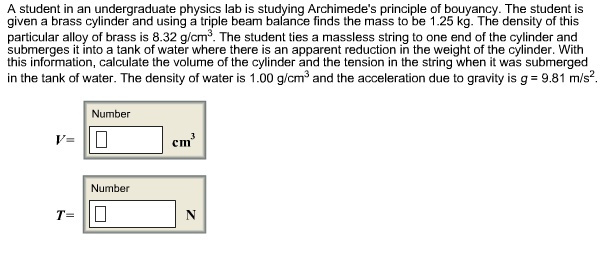# A student in an undergraduate physics lab is studying Archimede's principle of bouyancy. The student is given a brass cylinder and using á triple beam balance finds the mass to be 1.25 kg. The density of this particular alloy of brass is 8.32 g/cm3. The student ties a massless string to one end of the cylinder and submerges it into a tank of water where there is an apparent reduction in the weight of the cylinder. With this information, calculate the volume of the cylinder and the tension in the string when it was submerged in the tank of water. The density of water is 1.00 g/cm3 and the acceleration due to gravity is g 9.81 m/s2 Number V= Number T=

Questionhelp_outlineImage TranscriptioncloseA student in an undergraduate physics lab is studying Archimede's principle of bouyancy. The student is given a brass cylinder and using á triple beam balance finds the mass to be 1.25 kg. The density of this particular alloy of brass is 8.32 g/cm3. The student ties a massless string to one end of the cylinder and submerges it into a tank of water where there is an apparent reduction in the weight of the cylinder. With this information, calculate the volume of the cylinder and the tension in the string when it was submerged in the tank of water. The density of water is 1.00 g/cm3 and the acceleration due to gravity is g 9.81 m/s2 Number V= Number T= fullscreen

### Want to see this answer and more?

Experts are waiting 24/7 to provide step-by-step solutions in as fast as 30 minutes!*

*Response times may vary by subject and question complexity. Median response time is 34 minutes for paid subscribers and may be longer for promotional offers.
Tagged in
Science
Physics

### Fluid Mechanics